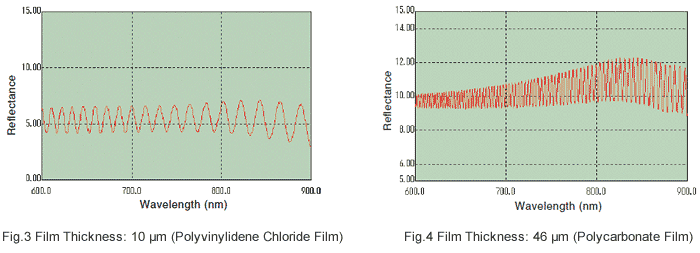# Film-Thickness Measurement

The thickness of a single-layer film can be measured easily using a spectrophotometer. Note, however, that this is possible only for film thickness in a range of approximately 0.3 to 60 μm, and that the refractive index of the film material is required for measurement.

First, I will explain the principle of film-thickness measurement relative to reflection measurement. When light strikes the film at a certain angle, there is interference between light reflected from the incident surface (A) and light reflected from the opposite surface (B),as shown in Fig.1, and the undulating interference spectrum shown in Fig.2 is created. Counting the number of peaks (or valleys) in the interference spectrum within a certain wavelength range makes it possible to calculate the film thickness using expression (1).

Here, "d" is the film thickness, "Δm" is the number of peaks in the wavelength range used for calculation, "n" is the refractive index, "θ" is the angle of incidence with respect to the sample, and "λ1" and "λ2" are the start and end wavelengths in the wavelength range used for calculation.

If film-thickness measurement software, which is sold as an option,is used, simply setting the wavelength range used for calculation and the refractive index makes it easy to calculate the film thickness, as shown in Fig.2. The "peak SD" value shown in Fig.2 is an indicator of the accuracy of the film-thickness calculation, and the calculation range is determined in accordance with this value.

As shown in Fig.3 and Fig.4, the pitch of the waveform is longer for thinner films and shorter for thicker films.If the film is on an opaque substrate, such as a wafer,reflection measurement is used to measure its thickness. If the film is on a transparent substrate, or if only the film itself is subjected to analysis, transmission measurement can be used to measure its thickness. In film-thickness measurement, it is necessary that the surface of the film is clean and has a mirror finish. Measurement is not possible for samples with rough surfaces.